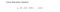# Logistic Regression in Python

Classification techniques are an essential part of machine learning & data mining applications. Approximately 70% of problems in Data Science are classification problems. There are lots of classification problems that are available, but the logistics regression is common and is a useful regression method for solving the binary classification problem. Another category of classification is Multinomial classification, which handles the issues where multiple classes are present in the target variable. For example, the IRIS dataset is a very famous example of multi-class classification. Other examples included fall under the category of classifying articles, blogs, & documents.

Logistic Regression is one of the most simple & commonly used Machine Learning algorithms for two-class classification. It’s easy to implement & can be used as the baseline for any binary classification problem. Its basic fundamental concepts are also constructive in deep learning. Logistic Regression describes & estimates the relationship between one and dependent binary variable and independent variables. It’s a statistical method for predicting binary classes. The outcome or target variable is dichotomous in nature which means that there are only two possible classes. For example, it can be used for cancer detection problems. It computes the probability of an event occurrence.

It is a special case of Linear Regression where the target variable is categorical in nature. It uses a log of odds as the dependent variable. Logistic Regression predicts the probability of occurrence of a binary event utilizing a log function.Where, y is dependent variable and X1, X2 … and Xn are explanatory variables.

Properties of Logistic Regression

• The dependent variable in Logistic Regression follows Bernoulli Distribution
• Estimation is done through maximum likelihood
• No R Square, Model fitness is calculated through Concordance, KS-Statistics

Linear Regression Vs. Logistic Regression

Linear Regression gives you a continuous output, but Logistic Regression provides a constant output. An example of the continuous output is house price and stock price. Examples of the discrete output is predicting whether a patient has cancer or not. Linear Regression is estimated using Ordinary Least Squares (OLS) while Logistic Regression is estimated using Maximum Likelihood Estimation (MLE) approach.

Maximum Likelihood Estimation Vs. Least Square Method

The MLE is a “likelihood” maximization method, while OLS is a distance-minimizing approximation method. Maximizing the likelihood function determines the parameters that are most likely to produce the observed data. From a statistical point of view, MLE sets the mean & variance as parameters in determining the specific parametric can be used for predicting the data needed in a normal distribution.

Ordinary Least Squares estimates are computed by fitting a regression line on given data points that has the minimum sum of the squared devotions (Least Square Error). Both are used to estimate the parameters of a linear regression model. MLE assumes a joint probability mass function, while OLS doesn’t require any stochastic assumptions for minimizing distance.

Types of Logistic Regression

• Binary Logistic Regression: the target variable has only two possible outcomes such as Spam or Not Spam, Cancer or No Cancer.
• Multinomial Logistic Regression: the target variable has three or more nominal categories such as predicting the type of Wine.
• Ordinal Logistic Regression: the target variable has three or more ordinal categories such as restaurant or product rating from 1 to 5.

Because of its efficient & straight forward nature, it doesn’t require high computation power, it’s easy to implement, easily interpretable, and used widely by data analysts/scientists. It also does not require scaling of features and it provides a probability score for observations.

Logistic Regression is not able to handle a large number of categorical features/variables. It is vulnerable to overfitting. Also, it can’t solve the non-linear problem with the Logistic Regression that is why it requires a transformation of non-linear features. Logistic Regression will not perform well with independent variables that are not correlated to the target variable & are very similar or correlated to each other.

References:

Written by